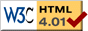# Analytic Solution for Single Spring

We seek a solution x(t) that satisfies the differential equation

x''(t) = − km x(t)

where

• x = position of the block
• t = time
• k = spring stiffness
• m = mass of the block

and the initial conditions

x(0) = x0   the position at time zero

x'(0) = v0   the velocity at time zero

For convenience we first define a new constant

c = √(k/m)

so the differential equation becomes

 x''(t) = −c2 x(t) (1)

We classify this differential equation as second order linear. It is second order because there is a second derivative: x'' . It is linear because all the functions or derivatives of x appear by themselves, only multiplied by a constant. To solve equations of this type, begin by assuming the solution is of the form

x(t) = e r t

where r is a constant, but possibly a complex number. The other constant e is the well-known mathematical exponential constant e = 2.718... which has nice properties for doing calculus. We plug this proposed solution into the differential equation (1) and get

r2 e r t = −c2 e r t

where we took the second derivative of e r t to get the left hand side. Since e r t is never equal to zero, we can divide it out of this equation to get

r2 = −c2

Since c > 0 the solution involves complex numbers and we have two values for r

r = ±c i

where i = √−1 . We then have two solutions

 x1(t) = e i c t (2a)
 x2(t) = e −i c t (2b)

Try plugging either of these solutions into the differential equation (1) and you will see that they work! Remember that i is just a constant number. Then the rules for differentiation of exponential functions give us:

x1'(t) = (i c)e i c t
x1''(t) = (i c) 2 e i c t = −c2 x1(t)

which matches the original differential equation (1). You might not know what it means to have an imaginary number as an exponent. If so, read on...

## Non-imaginary Solution

To return from the world of imaginary (complex) numbers we use Euler's formula:

e i z = cos z + i sin z

Applying Euler's formula to our solutions – equations (2a) and (2b) – we have

x1(t) = e i c t = cos(c t) + i sin(c t)

x2(t) = e i c t = cos(−c t) + i sin(−c t)

Using some basic trig identities we can simplify the second solution to be

x2(t) = cos(c t) − i sin(c t)

We still have the imaginary number i in these solutions. But now we can use a general property of linear differential equations:

a linear combination of solutions is also a solution

A linear combination of things is simply the sum of those things multiplied by constants. For example a linear combination of x, y, z could be

2 x − 0.333 y + 13 z

So we can form the following linear combinations which are also solutions but don't involve i :

12 x1(t) + 12 x2(t) = cos(c t)
i2 x1(t) + i2 x2(t) = sin(c t)

(remember that i is just a constant like any other number, so we can use it to form linear combinations). Now the general solution to the differential equation is given by a linear combination of the above solutions:

 x(t) = a cos(c t) + b sin (c t) (3)

where a, b are any constants. You can check that this still satisfies equation (1) by plugging it in.

## Satisfying the Initial Conditions

We still need to satisfy the initial conditions. Using equation (3) above, the first initial condition x(0) = x0 becomes

a cos(0) + b sin(0) = x0
a = x0

To evaluate the second initial condition x'(0) = v0 let's first calculate the derivative of our solution in equation (3).

x'(t) = −a c sin(c t) + b c cos(c t)

Now we can evaluate this at time t = 0 and use the second initial condition

a c sin(0) + b c cos(0) = v0
b c = v0
b = v0 c

Now we've found the particular a, b which match the initial conditions. So the particular solution is

 x(t) = x0 cos(c t) + v0 ⁄c sin(c t) (4)

## Check the Solution

We will check that equation (4) is the solution by plugging it into the differential equation (1).

 x''(t) = −c2 x(t) (1)

First we find the derivatives of equation (4).

x'(t) = −x0 c sin(c t) + v0 cos(c t)
x''(t) = −x0 c2cos(c t) − v0 c sin(c t)

This is the left hand side of equation (1). Now let's calculate the right hand side.

c2 x(t) = −c2 (x0 cos(c t) + v0 c sin(c t))
= −c2 x0 cos(c t) − v0 c sin(c t)

and so we see that both sides match – so our solution (4) satisfies the differential equation (1).

## Another Form of the Solution

The solution we found,

 x(t) = x0 cos(c t) + v0 ⁄c sin(c t) (4)

may be puzzling to you, because if you played with the simulation you will see that no matter what you do to it, it always exhibits a simple sine motion. Yet the above solution seems to be more complex than that. The answer to the puzzle is that the solution can be simplified to just a sine function using a trig identity. You get a sine function whose phase is shifted. Assuming v0 ≠ 0 , we get:

$$x(t) = \sqrt{x_0^2 + (v_0/c)^2} \; \sin \left( c t + \tan^{-1} \frac{c x_0}{v_0} \right)$$

So we can see that the behavior is always a simple sine motion. If the velocity is non-zero at the start, then the sine wave is phase shifted.

previous next